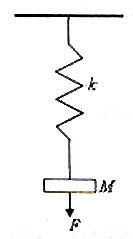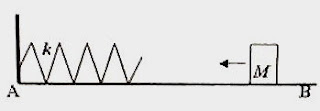## Pages

`“Life is like riding a bicycle.  To keep your balance you must keep moving.”–Albert Einstein`

## Tuesday, May 19, 2009

### AP Physics B&C - Work, Energy & Power - Multiple Choice Questions involving Springs

I am proud of the fact that I never invented weapons to kill

– Thomas Alva Edison

The essential points to be noted in the sections work, energy and power were discussed in the post dated 15th November 2008. Multiple choice questions in these sections were discussed in the posts dated 20th November 2008 and 30th November 2008. Two free response questions were discussed in the post dated 17th December 2008 You can access all those posts by clicking on the label ‘work’ or ‘energy’ below this post.

Today we will discuss a few multiple choice questions involving springs:(1) A spring of negligible mass and force constant (spring constant) k contained in a vertical barrel (Fig.) is free to move without friction vertically inside the barrel. A block of mass M, arranged at a height h from the top end of the unstretched spring, falls freely (without friction) from rest on the spring. The maximum compression x of the spring is given by the equation (acceleration due to gravity = g)

(a) mgx = ½ kx2

(b) mgh = ½ kx2

(c) mg (h+x) = ½ k[(h+x)2x2]

(d) mg (h+x) = ½ k[(h+x)2h2]

(e) mg (h+x) = ½ kx2

Since the mass M has moved down through a distance h+x when the spring undergoes the maximum compression x, the loss of gravitational potential energy by the mass M is mg (h+x).

The gain in the elastic potential energy by the spring in this condition is ½ kx2. The falling mass gains kinetic energy at the cost of gravitational potential energy. When it collides with the spring it transfers its kinetic energy to the spring and compresses the spring. When the spring is compressed to the maximum extent the entire kinetic energy of the mass is converted into elastic potential energy of the spring so that we have

mg (h+x) = ½ kx2

This equation gives the maximum compression x [Option (e)].

In the above question suppose the mass is initially resting on the spring. If the mass is depressed through a distance x and released, the spring will push the mass to a maximum height h (measured from the depressed position) given by the equation,mgh = ½ kx2

(2) A spring of negligible mass and of force constant (spring constant) 10 Nm–1 is suspended from the ceiling and it carries a mass M = 0.1 kg (Fig.). A downward force F is applied on this mass so that the spring suffers an additional stretch of 0.1 m. What is the work done by the force F? (Acceleration due to gravity, g = 10 ms–1).

(a) 0.14 J

(b) 0.15 J

(c) 0.2 J

(d) 0.39 J

(e) 0.1 J

The extension (x1) of the spring because of the suspended mass = Mg/k = (0.1×10)/10 = 0.1 m.

The work (W) done by the force F is equal to the increase in the total energy of the spring mass system. Note that the elastic potential energy of the spring has increased while the gravitational potential energy of the mass has decreased. Therefore we have

W = ½ k [(x1 + x2)2 x12] – mgx2

Or, W = ½ ×10×[0.04 – 0.01] – 0.01 = 0.14 J

(3) The elastic potential energy of a spring stretched by a small distance x is E. The spring is cut into two equal parts and one piece is stretched by the same distance x. The elastic potential energy of the stretched portion is

(a) E

(b) 2E

(c) 4E

(d) E/2

(e) E/4

When the length of a spring is halved, its spring constant is doubled. Its elastic potential energy for a given stretch x is quadrupled since the energy is directly proportional to the square of the stretch.

The correct option is (c).(4) The adjoining figure shows a block of mass M = 4 kg, moving with uniform velocity along a horizontal frictionless surface AB, towards a spring of force constant k = 100 Nm–1. One end of the spring is fixed to a rigid vertical wall. The block collides with the spring and produces in it a maximum compression of 0.1 m. What was the momentum of the block before the collision?

(a) 0.5 kg ms–1

(b) 2 kg ms–1

(c) 2.5 kg ms–1

(d) 4 kg ms–1

(e) 8 kg ms–1

Since the spring is compressed by 0.1 m, the elastic potential energy (which is ½ kx2 where x is the compression) of the spring is ½ ×100×(0.1)2 = 0.5 J.

Since the the block imparted this energy to the spring, the kinetic energy of the block was 0.5 J.

If p is the momentum of the block, we have

p2/2M = 0.5

Or, p2/(2×4) = 0.5 from which p = 2 kg ms–1

You can find similar useful questions (with solution) in this section here.